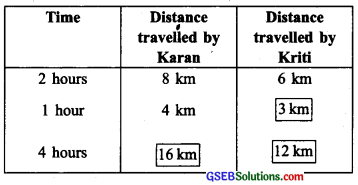# GSEB Solutions Class 6 Maths Chapter 12 Ratio and Proportion InText Questions

Gujarat Board GSEB Textbook Solutions Class 6 Maths Chapter 12 Ratio and Proportion InText Questions and Answers.

## Gujarat Board Textbook Solutions Class 6 Maths Chapter 12 Ratio and Proportion InText Questions

Try These (Page 245)

Question 1.
In class, there are 20 boys and 40 girls. What is the ratio of the number of boys to the number of girls?
Solution:
Number of boys = 20
Number of girls = 40
The ratio of the number of boys to the number of girls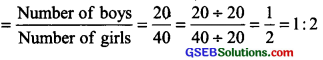Question 2.
Ravi walks 6 km in an hour while Roshan walks 4 km in an hour. What is the ratio of the distance covered by Ravi to the distance covered by Roshan?
Solution:
Distance covered by Ravi = 6 km
Distance covered by Roshan = 4 km
∴ The ratio of distance covered by Ravi to the distance covered by Roshan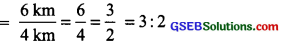Note:
(i) We denote ratio by the symbol ‘:’.
(ii) Ratio has no unit.
(iii) Two quantities can be compared only if they are in the same unit.
(iv) In a ratio the order of terms is very important. 2 : 3 and 3 : 2 are different ratios.Try These (Page 246)

Question 1.
Saurabh takes 15 minutes to reach school from his house and Sachin takes one hour to reach school from his house. Find the ratio of the time taken by Saurabh to the time taken by Sachin.
Solution:
Time taken by Saurabh = 15 minutes
Time taken by Sachin = 1 hour = 60 minutes
∴ The ratio of time taken by Saurabh to the time taken by Sachin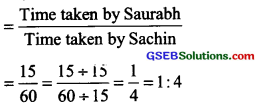[∵ HCF of 15 and 60 is 15]

Question 2.
The cost of the toffee is 50 paise and the cost of chocolate is ₹ 10. Find the ratio of the cost of toffee to the cost of chocolate.
Solution:
Cost of toffee = 50 paise
Cost of chocolate = ₹ 10 = 1000 paise
[∵ ₹ 1 = 100 paise]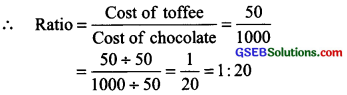[∵ HCF of 50 and 1000 is 50]

Question 3.
In school, there were 73 holidays in one year. What is the ratio of the number of holidays to the number of days in one year?
Solution:
Number of holidays = 73 days
Number of days in one year = 365 days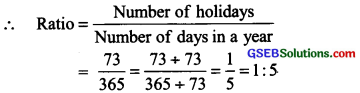[∵ HCF of 73 and 365 is 73]Try These (Page 248)

Question 1.
Find the ratio of the number of notebooks to the number of books in your bag.
Solution:
It is an activity, please do it yourself.

Question 2.
Find the ratio of the number of desks and chairs in your classroom.
Solution:
It is an activity, please do it yourself.

Question 3.
Find the number of students above twelve years of age in your class. Then find the ratio of the number of students with age above twelve years and the remaining students.
Solution:
It is an activity, please do it yourself.

Question 4.
Find the ratio of the number of doors and the number of windows in your classroom.
Solution:
It is an activity, please do it yourself.

Question 5.
Draw any rectangle and find the ratio of its length to its breadth.
Solution:
It is an activity, please do it yourself.Try These (Page 254)

Question 1.
Check whether the given ratios are equal, i.e. they are in proportion.
If yes, then write them in the proper form.
1. 1 : 5 and 3 : 15
2. 2 : 9 and 18 : 81
3. 15 : 45 and 5 : 25
4. 4 : 12 and 9 : 27
5. ₹ 10 to t 15 and 4 to 6
Solution:
1. 1 : 5 and 3 : 15
We have, 3 : 15 = $$\frac{3}{15}$$ = $$\frac{3\div 3}{15 \div 3}$$ =$$\frac{1}{5}$$ = 1 : 5
[∵ HCF of 3 and 15 is 3]
i.e., 1 : 5 and 3 : 15 are in proportion.
Thus, the proper form is 1 : 5 : : 3 : 15

2. 2 : 9 and 18 : 81
We have 18 : 81 = $$\frac{18}{81}$$ = $$\frac{18\div 9}{81 \div 9}$$ =$$\frac{2}{9}$$ = 2 : 9
[∵ HCF of 18 and 81 is 9]
i.e., 2 : 9 and 18 : 81 are in proportion.
Thus, the proper form is 2 : 9 : : 18 : 81

3. 15 : 45 and 5 : 25
We have, 15 : 45 = $$\frac{15}{45}$$ = $$\frac{15\div 15}{45 \div 15}$$ =$$\frac{1}{3}$$ = 1 : 3
[∵ HCF of 15 and 45 is 15]
Also, we have 5 : 25 = $$\frac{5}{25}$$ = $$\frac{5\div 5}{25 \div 5}$$ =$$\frac{1}{5}$$ = 1 : 5
[∵ HCF of 5 and 25 is 5]
∵ 1 : 3 ≠ 1 : 5
∴ 15 : 45 ≠ 5 : 25
i.e. 15 : 45 and 5 : 25 are a riot in proportion.

4. 4 : 12 and 9 : 27
We have, 4 : 12 = $$\frac{4}{12}$$ = $$\frac{4\div 4}{12 \div 4}$$ =$$\frac{1}{3}$$ = 1 : 3
[∵ HCF of 4 and 12 is 4]
Again we have,
We have, 9 : 27 = $$\frac{9}{27}$$ = $$\frac{9\div 9}{27 \div 9}$$ =$$\frac{1}{3}$$ = 1 : 3
[∵ HCF of 9 and 27 is 9]
∴ 4 : 12 = 9 : 27
or 4 : 12 and 9 : 27 are in proportion.
i.e., 4 : 12 : : 9 : 27 is the correct form.

5. ₹ 10 to ₹ 15 and 4 to 6
We have, ₹ 10 : ₹ 15 = $$\frac{10}{15}$$ = $$\frac{10\div 5}{15 \div 5}$$ =$$\frac{2}{3}$$ = 2 : 3
[∵ HCF of 10 and 15 is 5]
Again, we have, 4 : 6 = $$\frac{4}{6}$$ = $$\frac{4\div 2}{6 \div 2}$$ =$$\frac{2}{3}$$ = 2 : 3
[∵ HCF of 4 and 6 is 2]
Thus, ₹ 10 : ₹ 15 = 4 : 6.
or ₹ 10, ₹ 15, 4 and 6 are in proportion.
So, the correct form is ₹ 10 : ₹ 15 : : 4 : 6.Try These (Page 257)

Question 1.
Prepare five similar problems (on the unitary method) and ask your friends to solve them.
Solution:
It is project work. Please do it yourself.

Question 2.
Read the table and fill in the boxes.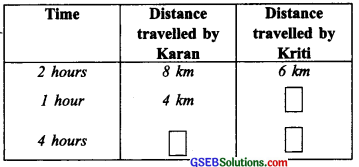Solution:
∵ Distance travelled by Kriti in 2 hours = 6 km
∴ Distance travelled by Kriti in 1 hour = $$\frac { 6 }{ 2 }$$ = 3 km
∵ Distance travelled by Karan in 1 hour = 4 km
∴ Distance travelled by Karan in 4 hours = 4 × 4 km = 16 km
∴ Distance travelled by Kriti in 1 hour = 3 km
∴ Distance travelled by Kriti in 4 hours = 3 × 4 km = 12km
Thus, the table is written as given below: